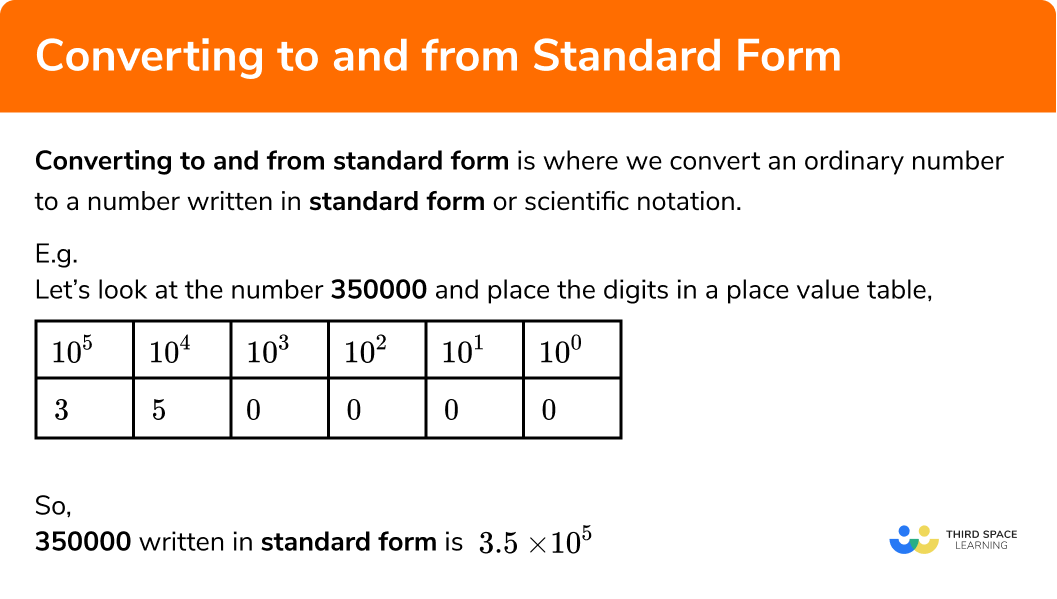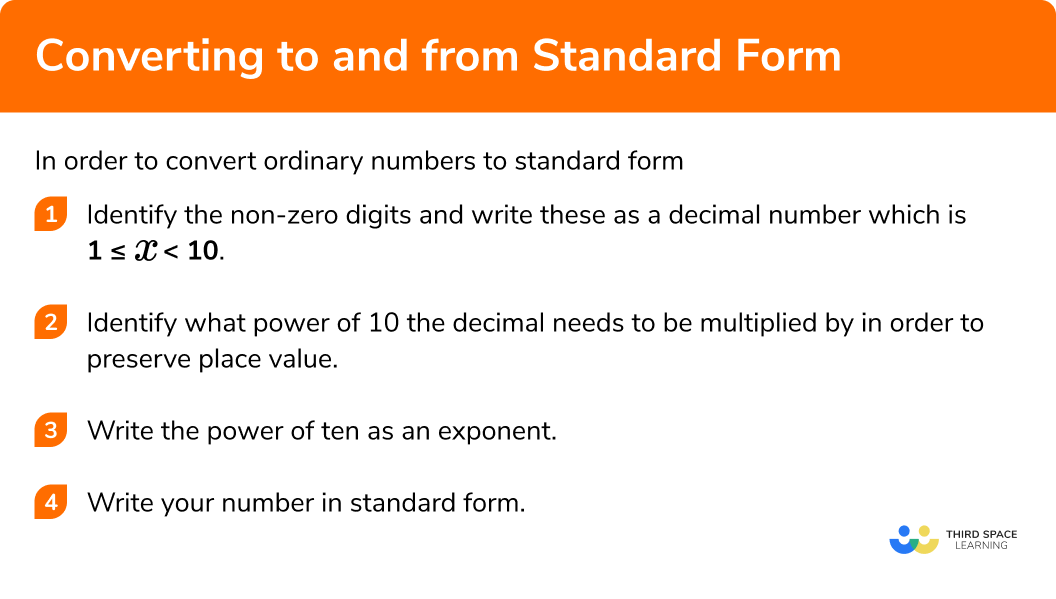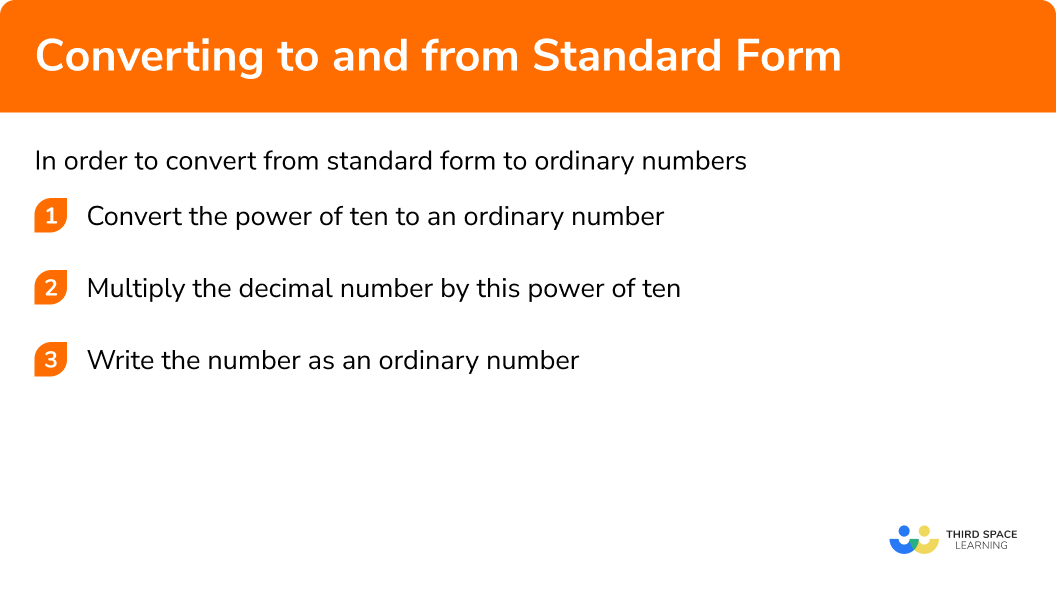GCSE Maths Number Standard Form

Converting to and from Standard Form

# Converting to and from Standard Form

Here we will learn how to convert to and from standard form, including how to adjust numbers to write them in standard form notation.

There are also converting to and from standard form worksheets based on Edexcel, AQA and OCR exam questions, along with further guidance on where to go next if you’re still stuck.

## What is converting to and from standard form?

Converting to and from standard form is where we convert an ordinary number to a number written in standard form or scientific notation.

Standard form is a way of writing very large or very small numbers by comparing the powers of ten.
Numbers in standard form are written in this format:

$a\times10^{n}$

Where a is a number 1\leq{a}\lt10 and n is an integer.

Converting between ordinary numbers and numbers in standard form can help us to compare numbers and interpret answers given in standard form on a calculator.
To do this we need to understand the place value of a number.

E.g.
Let’s look at the number 350000 and place the digits in a place value table:

\begin{aligned} &10^5 \quad \quad 10^4 \quad \quad 10^3\quad \quad 10^2 \quad \quad 10^1 \quad \quad 10^0 \\ &\; 3 \quad \quad \quad 5 \quad \quad \quad 0 \quad \quad \; \; \; 0 \quad \quad \; \; \; 0 \quad \quad \; \; \; 0 \end{aligned}

So 350000 written in standard form is:

$3.5\times10^{5}$

### What is converting to and from standard form?## How to convert ordinary numbers to standard form

In order to convert ordinary numbers to standard form:

1. Identify the non-zero digits and write these as a decimal number which is \pmb{ 1\leq{x}\lt10} .
2. In order to maintain the place value of the number, this decimal number needs to be multiplied by a power of ten.
3. Write the power of ten as an exponent.

### Explain how to convert ordinary numbers to standard form## Converting ordinary numbers to standard form examples

### Example 1: writing numbers in standard form with positive powers

Write this number in standard form:

480000

1. The non-zero digits need to be written in decimal notation.

The number needs to lie between 1\leq{x}\lt10

So the number will begin as 4.8….

2You now need to maintain the value of the number by multiplying that decimal by a power of ten.

$4.8 \times 100000 = 480000$

3Write that power of ten as an exponent.

$100000 = 10^{5}$

4Write your number in standard form.

$4.8\times10^{5}$

### Example 2: writing numbers in standard form with positive powers

Write this number in standard form:

5420000

The non-zero digits need to be written in decimal notation.

You now need to maintain the value of the number by multiplying that decimal by a power of ten.

Write that power of ten as an exponent.

Write your number in standard form.

### Example 3: converting a small number to standard form

Write 0.00081 in standard form.

Identify the non-zero digits and write these as a decimal number which is \pmb{1\leq{x}\lt10}.

Identify what power of ten the decimal needs to be multiplied by in order to preserve place value.

Write the power of ten as an exponent.

Write your number in standard form.

### Example 4: converting a small number to standard form

Write 0.00718 in standard form.

Identify the non-zero digits and write these as a decimal number which is \pmb{{1\leq{x}\lt10}.}

Identify what power of ten the decimal needs to be multiplied by in order to preserve place value.

Write the power of ten as an exponent.

Write your number in standard form.

## How to convert standard form to ordinary numbers

In order to convert from standard form to ordinary numbers:

1. Convert the power of ten to an ordinary number
2. Multiply the decimal number by this power of ten
3. Write your number as an ordinary number

### Explain how to convert standard form to ordinary numbers## Converting standard form to ordinary numbers examples

### Example 5: converting standard form to an ordinary number

Write 6.2\times10^4 as an ordinary number.

Write the exponent as a power of ten.

Multiply the decimal number by that power of ten.

### Example 6: converting standard form to an ordinary number

Write 1.9\times10^{-3} as an ordinary number.

Write the exponent as a power of ten.

Multiply the decimal number by that power of ten.

## How to adjust numbers to standard form

Sometimes we might have a number that looks like it is in standard form however the decimal number is not between 1 and 10,
E.g.
36103 or 0.2104.
In this case we need to adjust the number.

In order to adjust numbers to standard form:

1. Identify what power of ten the decimal number needs to be multiplied by so that the value is \pmb{1\leq{x}\lt10}.
2. Apply the inverse of this to the power of ten.

## Adjusting numbers to standard form examples

### Example 7: adjusting number in standard form

Write 48\times10^5 in standard form.

Identify what the first number needs to be multiplied or divided by so that it lies between \pmb{1\leq{x}\lt10}.

Apply the inverse operation to the power of ten.

Write your number in standard form.

### Example 8: adjusting numbers to standard form

Write 0.68\times10^{4} in standard form.

Identify what the first number needs to be multiplied or divided by so that it lies between \pmb{1\leq{x}\lt10}.

Apply the inverse operation to the power of ten.

Write your number in standard form.

### Example 9: adjusting numbers to standard form

Write 290\times10^{-4} in standard form.

Identify what the first number needs to be multiplied or divided by so that it lies between \pmb{1\leq{x}\lt10}.

Apply the inverse operation to the power of ten.

Write your number in standard form.

### Common misconceptions

• Writing a number with the incorrect power for a large or small number

This error is often made by counting the zeros following the first non zero digit for large numbers or zeros after the decimal point for small numbers, then writing this as the power, rather than considering the place value of the given number.

• Identifying incorrect place value with small numbers

In a number such as 0.000682, selecting the ‘2’ to determine the exponent rather than the ‘6’ which has a higher place value.
In standard form, this number would be 6.82 × 10^{-4}.

• Errors with negative numbers

When checking the standard form of a number, incorrectly adjusting the negative powers due to not applying negative numbers rules correctly.
E.g.
With small numbers, adding one to the power of 10^{-5} will result in 10^{-4} not 10^{-6}.

Converting to and from standard form is part of our series of lessons to support revision on standard form. You may find it helpful to start with the main standard form lesson for a summary of what to expect, or use the step by step guides below for further detail on individual topics. Other lessons in this series include:

### Practice standard form calculator questions

1. Write 270000 in standard form

27 \times 10^{4}2.7 \times 10^{4}2.7 \times 10^{5}0.27 \times 10^{6}The number between 1 and 10 here is 2.7.

\begin{aligned} 27000&=2.7 \times 100000\\\\ &=2.7 \times 10^{5} \end{aligned}

2. Write 0.00079 in standard form

7.9 \times 10^{-3}7.9 \times 10^{-4}7.9 \times 10^{-5}0.79 \times 10^{-4}The number between 1 and 10 here is 7.9.

\begin{aligned} 0.00079&=7.9 \times \frac{1}{10000}\\\\ &=7.9 \times 10^{-4} \end{aligned}

3. Write 6.1 \times 10^{4} as an ordinary number

6100061000.61 \times 10^{5}61000010^{4}=10000

Therefore,

\begin{aligned} 6.1 \times 10^{4} &= 6.1 \times 10000\\\\ &=61000 \end{aligned}

4. Write 3.8 \times 10^{-5} as an ordinary number

3800000.000380.00000380.00003810^{-5}=\frac{1}{100000}

Therefore,

\begin{aligned} 3.8 \times 10^{-5} &= 3.8 \times \frac{1}{100000}\\\\ &=3.8 \div 100000\\\\ &=0.000038 \end{aligned}

5. Write 84\times10^{2} in standard form

8408.4 \times 10^{2}8.4 \times 10^{3}8.4 \times 10This number is not in standard form as 84 is not between 1 and 10.

We need to divide 84 by 10 and, to compensate, multiply 10^2 by 10 , increasing the power by 1.

This gives us

8.4 \times 10^{3}

6. Write 0.92\times10^{-5} in standard form

9.2 \times 10^{-4}9.2 \times 10^{-5}9.2 \times 10^{-6}0.92 \times 10^{-4}This number is not in standard form because 0.92 is not between 1 and 10.

We need to multiply 0.92 by 10 and, to compensate, divide 10^{-5} by 10 , decreasing the power by 1.

This gives us

9.2 \times 10^{-6}

### Standard form calculator GCSE questions

1.

(a)  Write 8.23\times10^{-6} , as an ordinary number.

(b)  Write the number 0.00702 in standard form.

(2 marks)

(a)  0.00000823

(1)

(b)  7.02\times10^{-3}

(1)

2.

(a)  The population of the USA is 3.3\times10^{8} , rounded to two significant figures.

Write this distance as an ordinary number.

(b) The population of Washington DC is 690000 rounded to two significant figures.

Write this number in standard form.

(2 marks)

(a)  3 30000000 km

(1)

(b)  6.9\times10^{5}km

(1)

3.   Put the below numbers in order. Start with the smallest number.

(2 marks)

Converting all of the numbers to the same form or standard notation for comparison or 3 of the four numbers ordered correctly.

(1)

(1)

## Learning checklist

You have now learned how to:

• Convert ordinary numbers standard form
• Convert standard form to ordinary numbers
• Adjust numbers to standard form notation

## Still stuck?

Prepare your KS4 students for maths GCSEs success with Third Space Learning. Weekly online one to one GCSE maths revision lessons delivered by expert maths tutors.

Find out more about our GCSE maths tuition programme.